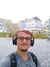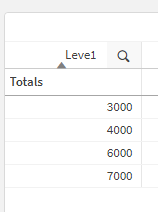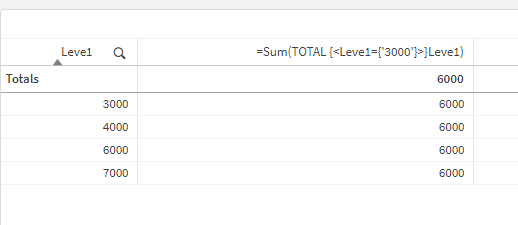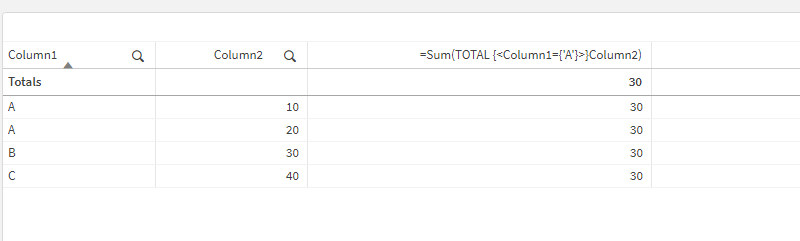# New to Qlik Sense

If you’re new to Qlik Sense, start with this Discussion Board and get up-to-speed quickly.

Announcements
QlikWorld 2023, a live, in-person thrill ride. Save \$300 before February 6: REGISTER NOW!
cancel
Showing results for
Did you mean:Contributor III

## Total values in table

Hi Gurus,

I need to get the totals of some valies in the Column

ex:
I got a table with couple of columns and In one column I have this values:

Column1  column2
A           20
B          30
C         40
A         10

Now SUm of column2 is 100

but i need to show only sum of A 20+10 = 30

Thanks,
Kumar

Labels (5)

• ### Visualization

1 Solution

Accepted SolutionsSpecialist

Hello,

I am not 100% sure that I understood your requirement.

You have stated: "now I want sum where level 1 =3000 i.e 6000", from which I understand that you want to sum all the values of field "Leve1" where the amount is equal to "3000". Therefore, based on the given dataset you should get the outcome "6000".

Then you have stated: "I  need to show in a table .", which I am not sure how the data should appear on the final table.

1. Based on the new details I have loaded the dataset and added "Leve1" as dimension in the table:2. Now I have added a measure expression:  =Sum(TOTAL {<Leve1={'3000'}>}Leve1) and the outcome is:It only sum up all the values of field Leve1 where the value is 3000 and displays on the table, so ideally it covers what you need.

However, in case I am still missing some details, then I would recommend:

1. Create a simple table with the data in EXCEL and share with us a screenshot
2. Then create another table that will represent the expected outcome and share again the screenshot
3. This will allow us to understand exactly the use case scenario

Help users find answers! Don't forget to mark a solution that worked for you! 🙂
4 RepliesSpecialist

Hello,

Perhaps you can use set analysis? For example:

You can use the expression: =Sum(TOTAL {<Column1={'A'}>}Column2) and the output is:As you can see only the number 30 is presented, which is the sum of all A values from Column2.

I hope that this information was helpful. In case I have misunderstood the use case scenario, please elaborate in details by providing additional information. However, if it has helped you resolve the issue, addressed your concerns or at least pointed you in the right direction, please mark it as Accepted Solution to give further visibility to other community members.

Help users find answers! Don't forget to mark a solution that worked for you! 🙂Contributor III
Author

Sorry Andrei,

I explained the scenario in a wrong way ..plz bear with me as I am new to QS

I have a Column Leve 1

Leve1

3000

4000

3000

6000

7000

now I want sum where level 1 =3000 i.e 6000 I  need to show in a table .

KumarSpecialist

Hello,

I am not 100% sure that I understood your requirement.

You have stated: "now I want sum where level 1 =3000 i.e 6000", from which I understand that you want to sum all the values of field "Leve1" where the amount is equal to "3000". Therefore, based on the given dataset you should get the outcome "6000".

Then you have stated: "I  need to show in a table .", which I am not sure how the data should appear on the final table.

1. Based on the new details I have loaded the dataset and added "Leve1" as dimension in the table:2. Now I have added a measure expression:  =Sum(TOTAL {<Leve1={'3000'}>}Leve1) and the outcome is:It only sum up all the values of field Leve1 where the value is 3000 and displays on the table, so ideally it covers what you need.

However, in case I am still missing some details, then I would recommend:

1. Create a simple table with the data in EXCEL and share with us a screenshot
2. Then create another table that will represent the expected outcome and share again the screenshot
3. This will allow us to understand exactly the use case scenario

Help users find answers! Don't forget to mark a solution that worked for you! 🙂Contributor III
Author

Thanks.Tags
Community Browser